## Home# Boolean Algebra

Outline

### What is Boolean Algebra

Boolean algebra is a special branch of algebra which is mostly used in digital electronics. Boolean algebra was invented in the year of 1854, by an English mathematician George Boole.

Boolean algebra is a method of simplifying the logic circuits (or sometimes called as logic switching circuits) in digital electronics.

So it is also called as “Switching algebra”. We can represent the functioning of logic circuits by using numbers, by following some rules, which are well known as “Laws of Boolean algebra”.

We can also make the calculations and logical operations of the circuits even faster by following some theorems, which are known as “Theorems of Boolean Algebra”. A Boolean function is a function which represents the relation between the input and output of a logic circuit.

Boolean logic allows only two states of the circuit, such as True and False. These two states are represented by 1 and 0, where 1 represents the state “True” and 0 represents the state “False”.

The most important thing to remember in Boolean algebra is that it is very much different than regular mathematical algebra and its methods. Before learning about the Boolean algebra, lets us know about the history of Boolean algebra and its invention and development.

### History of Boolean Algebra

As mentioned earlier, Boolean algebra is invented in the year of 1854, by an English mathematician George Boole. He first stated the idea of the Boolean algebra in his book “An Investigation of the Laws of Thought”.

After this, the Boolean algebra is well known as the perfect way for representing the digital logic circuits.

In the late 19th century, scientists Jevons, Schroder and Huntington used this concept for modernized concepts. And in the year of 1936, M.H.Stone proved that the Boolean algebra is ‘isomorphic’ to the sets (A functional area in mathematics).

In 1930s , a scientist named, Claude Shannon developed a new type algebra method as “Switching Algebra” by using the Boolean algebra concepts, for studying the switching circuits.

The logic synthesis of the modern electronic automation tools is efficiently represented by using Boolean functions known as “Binary Decision Diagrams”.

Boolean algebra allows only two states of a logic circuit, as True and False or High and Low or Yes and No or Open and Close or 0 and 1.

#### Boolean Expressions

These are similar to that of the mathematical expression. The Boolean expressions are formed by combining the logical variables by using the logical operators. For example

• X + Y
• X + Y + X Z’
• X’ + Y’

### Postulates of Boolean Algebra

There are some basic laws and rules that the Boolean algebraic system must follow. They are known as “Laws of Boolean algebra”.

Properties of 1 and 0

0 + X = X

1 + X = 1

0 . X = 0

1 . X = X

X + 0 = X

X . 1 = X

X + X = X

X . X = X

X.0 = 0

X + 1 = 1

X + X’ = 1

X . X’ = 0

X + Y = Y + X

X . Y = Y . X

#### Distributive Law

X. (Y + Z) = X.Y + X.Z

X + (Y.Z) = (X + Y).(X + Z)

#### Associative Law

X + (Y + Z) = (X + Y) + Z (OR Associative)

X .(Y.Z) = (X . Y) Z (AND Associative)

#### Absorption Laws

X + X.Y = X (OR Absorptive)

X .(X + Y) = X (AND Absorptive)

X + X’.Y = X + Y

X.(X’ + Y) = X.Y

#### Combining Law

X . Y + X . Y’= X

(X + Y) (X +Y’) = X

(X’)’ = X

#### Consensus Laws

X.Y + X’.Z + YZ = X.Y + X’.Z

(X + Y).(X’ + Z).(Y + Z) = (X + Y).(X’ + Z)

Boolean ExpressionDescriptionEquivalent switching circuitBoolean Law
X + 1 = 1X in parallel with closed = "CLOSED"Annulment
X + 0 = XX in parallel with open = "X"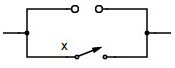Identity
X . 1 = XX in series with closed = "X"Identity
X . 0 = 0X in series with open = "OPEN"Annulment
X + X = XX in parallel with X = "X"Idempotent
X . X = XX in series with X = "X"Idempotent
NOT X = XNOT NOT X (Double negative) = "X"Double Negation
X + X = 1X in parallel with NOT X = "CLOSED"Complement
X . X = 0X in series with NOT X  =  "OPEN"Complement
X+Y = Y+XX in parallel with Y =Y in parallel with X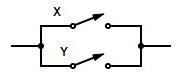Commutative
X.Y = Y. XX in series with Y =Y in series with X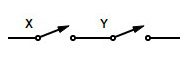Commutative
(X +Y) = X.YInvert and replace OR with ANDDe Morgans Theorem
(X.Y) = X+YInvert and replace AND with ORDe Morgans Theorem

### Boolean Logical Operations

In general mathematics, we represent the mathematical operations between algebraic variables by using mathematical operators like +, -, *, /. Similarly, in Boolean algebra, we represent the Boolean operations by using logical operators like AND, OR, NOT operations.

The basic Boolean arithmetic operations are of 3 types. They are AND operation, OR operation and NOT operation. Always, we represent the Boolean operation in capital letters.

Representing the operations in lower case letters is a wrong way. Let’s discuss about the Boolean arithmetic operations.

#### Complement (NOT Function)

Complement means ‘The reversal or inverse or opposite value’. Boolean algebra supports the complementation law. For example, if the variable is 1, then its complement will be 0.

Similarly, if the variable is 0, then its complement will be 1. The complement variable is represented by a ‘bar’ on the variable.

The complement operation is also known as NOT operation. NOT gate performs the Boolean complement operation.

If X = 1, then X ̅ = 0

If X = 0, then X ̅ = 1

The complemented output X ̅ can be read as X – bar or X – not. We also represent the complimented variable by a ‘prime’ symbol (’) like X’.

The logic symbol for the NOT gate is shown below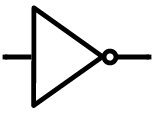OR functioning means the Boolean addition of binary numbers. It produces the sum of the two binary numbers such as

0 + 0 = 0

0 + 1 = 1

1 + 0 = 1

1 + 1 =1

Boolean OR operation is explained by using the OR gate and parallel switch contacts.

For 0 + 0 = 0For 0 + 1 = 1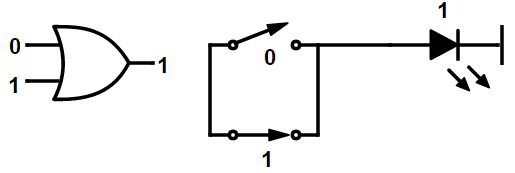For 1 + 0 = 1For 1 + 0 = 1For 1 + 1 = 1The important thing to remember in Boolean algebra is that there is no direct mechanism for adding the negative numbers. This means there is no possibility for the direct subtraction in Boolean algebra. Subtraction is nothing but the “Compounded Addition”. For example, 4 – 2 is same as the 4 + (-2).

#### Multiplication (AND Function)

AND functioning means the Boolean multiplication of binary numbers. It produces the product of the two binary numbers such as

0 . 0 = 0

0 . 1 = 0

1 . 0 = 0

1 . 1 = 1

Boolean AND operation is explained by using the AND gate and series switch contacts.

For 0 . 0 = 0For 0 . 1 = 0For 1 . 0 = 0For 1 . 1 = 1It is an important thing to remember in Boolean algebra is that there is no direct mechanism for the division of two numbers. Division is nothing but the “Compounded multiplication”.

### Simplification of Boolean functions

By using the Boolean theorems and Boolean laws, we can simplify the Boolean expressions, by which we can reduce the required number of logic gates to be implemented. We can simplify the Boolean function by using two methods,

1. The algebraic method – by using identities (Boolean laws).
2. The graphical method – by using Karnaugh Map method

The K-map method is very easy to simplify a function than using identities. If n is the number of variables, then the K- map consists of 2n cells and there will be no similar value for any of the two adjacent rows of columns.